# 清除格值

## 格值的置空与序表的 reset

 A 1 =file("Order_Appliances.txt").import@t() 2 =file("Order_Foods.txt").import@t() 3 =A1|A2 4 =A3.top(200;-Amount).new(ID, PName,Type,Date,Amount) 5 =A4.sort(PName)

A1中的序表为Order_Appliances.txt中的订单记录：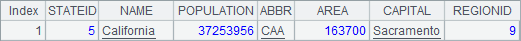A2的序表为Order_Foods.txt中的订单记录：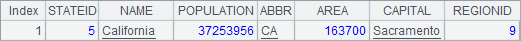A3将前面两个序表中的记录简单合并在一起，为下一步的过滤做准备。

A4中选出销售额前200名的订单记录，并从中选出需要的字段生成新序表。由于这里需要按销售额从大到小排列，因此在top() 函数中，Amount前面有负号，此时获得的结果是按照销售额降序排序的：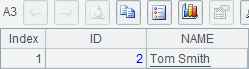A5中，按照需要，将销售额前200名的订单记录按照商品名称排序：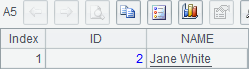A B C 1 =file("Order_Appliances.txt").import@t() 2 =file("Order_Foods.txt").import@t() 3 =A1|A2 >A1.reset() >A2=null 4 =A3.top(200;-Amount).new(ID, PName,Type,Date,Amount) >A3=null 5 =A4.sort(PName) >A4=null

B3中的T.reset() 函数则有所不同，它会清除掉序表中的所有记录，但仍保留其数据结构，执行完B3A1的值如下：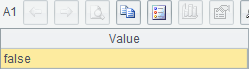## 使用 clear 语句清除格值

 A B 1 =file("Order_Appliances.txt").import@t() 2 =file("Order_Foods.txt").import@t() =A1|A2 3 =B2.top(200;-Amount).new(ID, PName,Type,Date,Amount) 4 =A3.sort(PName) 5 >A1=null >A2=null 6 >B2=null >A3=null

 A B 1 =file("Order_Appliances.txt").import@t() 2 =file("Order_Foods.txt").import@t() =A1|A2 3 =B2.top(200;-Amount).new(ID, PName,Type,Date,Amount) 4 =A3.sort(PName) 5 clear A1,A2,B2,A3

 A B 1 =file("Order_Appliances.txt").import@t() 2 =file("Order_Foods.txt").import@t() =A1|A2 3 =B2.top(200;-Amount).new(ID, PName,Type,Date,Amount) 4 =A3.sort(PName) 5 clear A1:B3

A5clear命令中，A1:B3表示A1B3格所在的矩形区域，即A1,B1,A2,B2,A3,B3所组成的区域，clear命令会清除所有这些单元格的格值。在clear命令中，单个单元格和单元格区域的写法可以同时使用。如A5中的clear命令也可以写为clear A1:B2,A3，效果是相同的。

 A B 1 [Order_Appliances.txt,Order_Foods.txt] =*A1.len() 2 for A1 =file(A2).import@t() 3 =B2.top(100;-Amount) 4 =B3.sort(PName) 5 >B1(#A2)=B4 6 =B1.merge(PName) 7 =A6.new(ID, PName,Type,Date,Amount)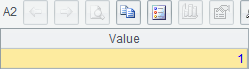A B 1 [Order_Appliances.txt,Order_Foods.txt] =*A1.len() 2 for A1 =file(A2).import@t() 3 =B2.top(100;-Amount) 4 =B3.sort(PName) 5 >B1(#A2)=B4 6 =B1.merge(PName) 7 =A6.new(ID, PName,Type,Date,Amount) clear A1:B1,A2:,A6

B7clear命令中，A2:的写法和单元格区域类似，但没指定区域的结束格。这样的写法表示清除以A2为主格的区块中所有单元格的值，即清除第2~5行的所有单元格的格值。执行后，除了A7中的结果，其它单元格中的格值都被清除。